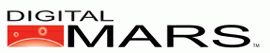D Programming Language 1.0

Last update Mon Dec 31 10:53:28 2012

# std.intrinsic

These functions are built-in intrinsics to the compiler.

Intrinsic functions are functions built in to the compiler, usually to take advantage of specific CPU features that are inefficient to handle via external functions. The compiler's optimizer and code generator are fully integrated in with intrinsic functions, bringing to bear their full power on them. This can result in some surprising speedups.

License:
Public Domain

Authors:
Walter Bright

Source:
std/intrinsic.d

int bsf(size_t v);
Scans the bits in v starting with bit 0, looking for the first set bit.

Returns:
The bit number of the first bit set. The return value is undefined if v is zero.

int bsr(size_t v);
Scans the bits in v from the most significant bit to the least significant bit, looking for the first set bit.

Returns:
The bit number of the first bit set. The return value is undefined if v is zero.

Example:
``` import std.stdio;
import std.intrinsic;

int main()
{
uint v;
int x;

v = 0x21;
x = bsf(v);
writefln("bsf(x%x) = %d", v, x);
x = bsr(v);
writefln("bsr(x%x) = %d", v, x);
return 0;
}
```

Output:
bsf(x21) = 0
bsr(x21) = 5

int bt(uint* p, size_t bitnum);
Tests the bit.

int btc(uint* p, size_t bitnum);
Tests and complements the bit.

int btr(uint* p, size_t bitnum);
Tests and resets (sets to 0) the bit.

int bts(uint* p, size_t bitnum);
Tests and sets the bit.

Params:
 uint* p a non-NULL pointer to an array of size_ts. index a bit number, starting with bit 0 of p, and progressing. It addresses bits like the expression: ```p[index / (size_t.sizeof*8)] & (1 << (index & ((size_t.sizeof*8) - 1))) ```

Returns:
A non-zero value if the bit was set, and a zero if it was clear.

Example:
```import std.stdio;
import std.intrinsic;

int main()
{
size_t array;

array = 2;
array = 0x100;

writefln("btc(array, 35) = %d", <b>btc</b>(array, 35));
writefln("array = :x%x, :x%x", array, array);

writefln("btc(array, 35) = %d", <b>btc</b>(array, 35));
writefln("array = :x%x, :x%x", array, array);

writefln("bts(array, 35) = %d", <b>bts</b>(array, 35));
writefln("array = :x%x, :x%x", array, array);

writefln("btr(array, 35) = %d", <b>btr</b>(array, 35));
writefln("array = :x%x, :x%x", array, array);

writefln("bt(array, 1) = %d", <b>bt</b>(array, 1));
writefln("array = :x%x, :x%x", array, array);

return 0;
}
```

Output:
```btc(array, 35) = 0
array = :x2, :x108
btc(array, 35) = -1
array = :x2, :x100
bts(array, 35) = 0
array = :x2, :x108
btr(array, 35) = -1
array = :x2, :x100
bt(array, 1) = -1
array = :x2, :x100
```

uint bswap(uint v);
Swaps bytes in a 4 byte uint end-to-end, i.e. byte 0 becomes byte 3, byte 1 becomes byte 2, byte 2 becomes byte 1, byte 3 becomes byte 0.

ubyte inp(uint port_address);
ushort inpw(uint port_address);
uint inpl(uint port_address);
Reads I/O port at port_address.

ubyte outp(uint port_address, ubyte value);
ushort outpw(uint port_address, ushort value);
uint outpl(uint port_address, uint value);
Writes and returns value to I/O port at port_address.